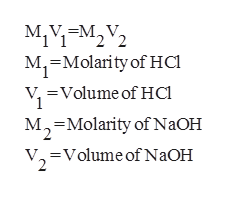# Calculate the concentration (in molarity) of an NaOH solution if 25.0 mL of the solution is needed to neutralize 17.1 mL of a 0.417 M HCl solution.

Question
51 views

Calculate the concentration (in molarity) of an NaOH solution if 25.0 mL of the solution is needed to neutralize 17.1 mL of a 0.417 M HCl solution.

check_circle

Step 1

The balanced equation will be:

Step 2

According to the formulahelp_outlineImage TranscriptioncloseM1VEM22 М, M, Molarity of HCl =Volume of Hc M-Molarity of NaOH 2 Volume of NaOH fullscreen
Step 3

Given,

Molarity of HCl is 0.417M.

Volume of HCl is 17.1 millilitre.

Molarity of NaOH is ?

Volume of NaOH is...

### Want to see the full answer?

See Solution

#### Want to see this answer and more?

Solutions are written by subject experts who are available 24/7. Questions are typically answered within 1 hour.*

See Solution
*Response times may vary by subject and question.
Tagged in

### General Chemistry